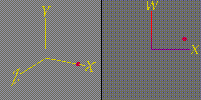# Rotation of a single pointClick on this picture to see a movie of a point rotating in four dimensions. You should see two panels each showing a set of axes and a point. Let's call the panel on the left the X_Y_Z_hyperplane, and the panel on the right the X_W_plane.

Notice what is happening in the X_Y_Z_hyperplane-- the point is moving along the x axis.

What's going on?

We are watching several projections of a point moving in four dimensional space. Unfortunately, we can't show all four dimensions in the picture at once, so we show more than one view, and piece together the information in both panels to understand what is going on in 4-dimensions.

The point in the picture above is shown starting out at approximately (x=.7, y=0, z=0, w=.3). It is moving in a circle in the X_W_plane.

The movement of the point in the X direction can been seen in the projection into the X_Y_Z_hyperplane, but in that panel its movement in the W direction has been hidden (or "projected out").

Everything has been color-coded according to height in the w direction, so that when the w coordinate of the point is high, the point is colored red, and when it is low, the point is colored blue.

Although the point looks like its just moving back and forth in the X_Y_Z_hyperplane, we can see from the changing color that it is simultaneously moving around in the W direction. This is our first example of motion in a four dimensional space.

```Olaf Holt  3/14/94
```